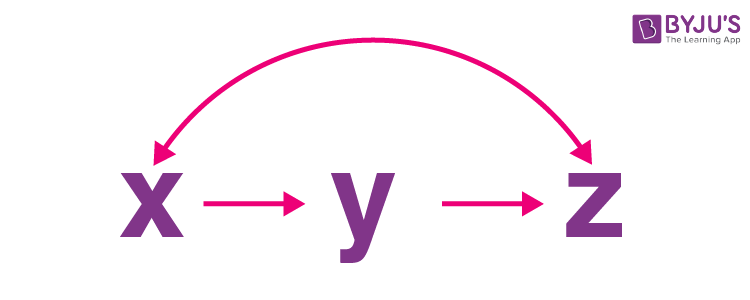# Transitive Property

In Mathematics, a transitive relation is defined as a homogeneous relation R over the set A, where the set contains the elements such as x, y and z, such that R relates x to y and y to z, then R also relates x to z. In this article, we will learn the definition of “Transitive Property”, transitive property of equality, inequality, congruence and many solved examples with complete explanation.

## What is Transitive Property?

A property is called transitive property, if x, y and z are the three quantities, and if x is related to y by some rule, and y is related to z by the same rule, then we can say x is related to z by the same rule.The transitive property can be used for relating the quantities if we have three or more than three quantities. The transitive property can be applied to algebraic expressions, numbers, and different geometrical concepts, in which the quantities are related by some rule.

## Transitive Property of Equality

The transitive property of equality formula is given as follows:

If x = y and y = z, then x = z.

Where x, y and z belongs to the same category elements.

For example, if “x” represents the measurement of a line segment, then y and z should represent the measurement of the line segment.

The transitive property is extended to the transitive property of inequalities as well as the transitive property of congruence. Now, let us discuss the transitive property of inequalities and transitive property of congruence one by one.

## Transitive Property of Inequality

We can also apply the transitive property to the inequalities. We know that the different inequalities in Maths are less than (<), greater than (>), less than or equal to (≤) and greater than or equal to (≥). Similar to the transitive property of equality, we can formulate the transitive property of inequalities, which are given below:

For any real numbers, x, y and z, the transitive property of inequalities states that:

• If x < y and y < z, then x < z.
• If x > y and y > z, then x > z.
• If x ≤ y and y ≤ z, then x ≤ z.
• If x ≥ y and y ≥ z, then x ≥ z.

In case, if any of the relations mentioned above, where either of the premises is a strict inequality, then the final result should also be in strict inequality.

For example, if x ≤ y and y < z, then x < z.

## Transitive Property of Congruence

The transitive property of congruence states that “ if two shapes are congruent to the third shape, then all the shapes are congruent to each other.

Let us consider three triangles,

First triangle = ΔABC

Second triangle = ΔPQR

Third Triangle = ΔXYZ.

Thus, the transitive property of congruence is given as follows:

If ΔABC ≅ ΔPQR and ΔPQR ≅ ΔXYZ, then ΔABC ≅ ΔXYZ

It means that the triangle ABC is congruent to triangle PQR, and triangle PQR is congruent to triangle XYZ, then we can say that the triangle ABC is congruent to the triangle XYZ.

### Transitive Property Examples

Go through the following examples to understand the concept of transitive property:

Example 1:

Find the value of x, where x = y and y = 5.

Solution:

Given: x = y and y = 5.

By using the transitive property of equality (i.e) if a = b and b = c, then a = c, we can find the value of x.

If x =y and y = 5, then x = 5.

Hence, the value of x is 5.

Example 2:

Determine the value of “a” using the transitive property: a+b = c and c = 3b

Solution:

Given equations:

a + b = c … (1)

c = 3b … (2)

By using the transitive property, equation (1) and (2) is written as:

a + b = 3b

a = 3b – b

a = 2b.

Therefore, the value of a is 2b.

### Practice Problems

Solve the following problems using the transitive property:

1. Find the value of a, if a = b and b = 86.
2. Find the value of ∠R, if ∠P = ∠Q and ∠Q = ∠R, where ∠P = 60°.
3. The weight of the apple is the same as the weight of the orange, and the weight of orange is equal to the weight of the grapes. Find the weight of the apple, if the weight of the grapes is equal to 2 kilograms.

## Frequently Asked Questions on Transitive Property

Q1

### What is meant by transitive property?

The transitive property states that “if two quantities are equal to the third quantity, then we can say that all the quantities are equal to each other”.

Q2

### How do you represent the transitive property?

If a, b and c are the three quantities and belong to the same category elements, then the transitive property is represented as follows:
If a = b and b = c, then a = c.

Q3

### What is the transitive property of inequalities?

If a, b and c are the real numbers, then the transitive property of inequality is given as:
If a < b and b < c, then a < c.
Transitive property can also be applied to other inequalities, such as >, ≤ and ≥.

Q4

### What is the value of x, if x = y and y = 9?

By using the transitive property, if x = y and y=9, then x = 9.
Therefore, the value of x is 9.

Q5

### When do we use the transitive property?

The transitive property is used, when we have three or more than three quantities, which are of the same category and related by some rule.